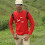# Implementation of Bresenham's line drawing algorithm [CS1255 - Graphics and Multimedia Lab]

AIM:

To write a "C++" program for the implementation of Bresenham's line drawing algorithm in CS1255 - Graphics and Multimedia Lab.

SOURCE CODE:

#include<iostream.h>
#include<conio.h>
#include<math.h>
#include<graphics.h>
class lines
{
private:
int dx,dy,x,y,xend,p,xa,ya,xb,yb;
public:
void getdata();
void line();
void disdata();
};
void lines::getdata()
{
cout<<"\n\tEnter xa and ya :";
cin>>xa>>ya;
cout<<"\n\tEnter xb and yb :";
cin>>xb>>yb;
}
void lines::line()
{
dx=abs(xa-xb);
dy=abs(ya-yb);
p=2*dy-dx;
if(xa>xb)
{
x=xb;
y=yb;
xend=xa;
}
else
{
x=xa;
y=ya;
xend=xb;
}
}

void lines::disdata()
{
putpixel(x,y,1);

while(x<xend)
{
x=x+1;
if(p<0)
{
p=p+2*dy;
}
else
{
y=y+1;
p=p-2*dy-2*dx;
}
putpixel(x,y,1);
}
}
void main()
{
lines l;
clrscr();
int gd=DETECT,gm;
initgraph(&gd,&gm,"C:/TC/BGI");
l.getdata();
l.line();
l.disdata();
getch();
closegraph();
}

OUTPUT:

Previous
Next Post »

dietzwallace
AUTHOR

Tried it on desktop but the line is not displaying.Jebastin
AUTHOR

Actually its working fine. Just now checked again.

1)Check the path C:/TC/BGI
Give as in your system. If you are having TC in other drive means, Change it to that.

2)It displays a blue line. You might not recognized that.

3)Give big values. Because its pixel value of starting and end point.
For example, Give xa=10, ya=20, xb=50, yb=60.

Here in this website, All the programs were output verified by me. So no problem with the coding.